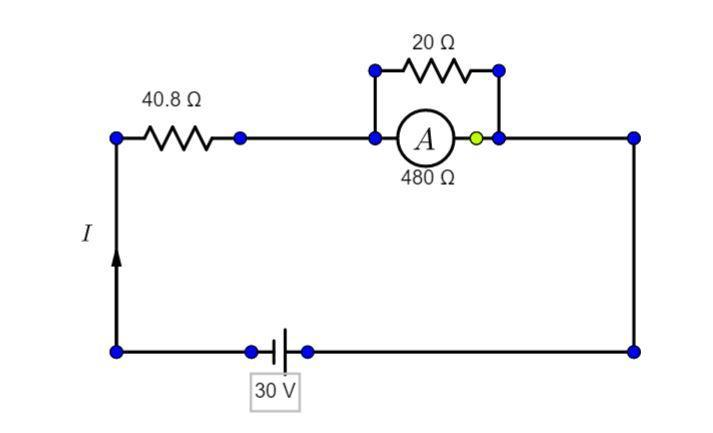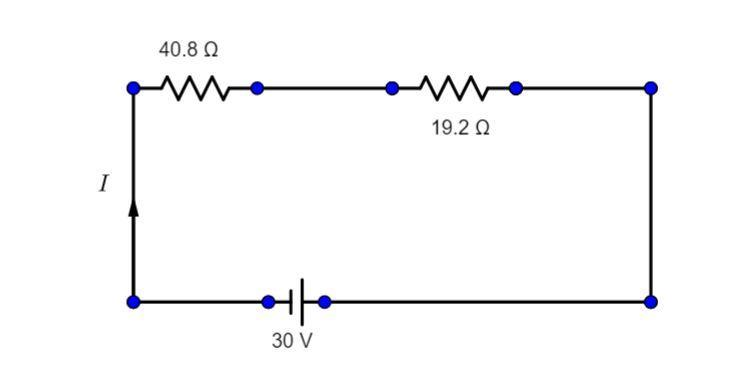QuestionAnswers

# A circuit contains an ammeter, a battery of 30V and a resistance 40.8ohm all connected in series. If the ammeter has a coil of resistance 480ohm and a shunt of 20ohm, the reading in the ammeter will be:(A). 1A(B). 0.5A(C). 0.25A(D). 2AHint: The ammeter is in series with the 40.8 ohm resistance, and ammeter is in parallel to the shunt resistance. We know that ohm’s law states that voltage or potential difference in a circuit is completely proportional to the current and resistance of the circuit.

Complete step-by-step solution -Now, According to the problem
If, we consider resistance of ammeter to be ${{R}_{1}}$ and shunt resistance as ${{R}_{2}}$.
And both of them are in parallel to each other then we can find the equivalent resistance of that circuit.
${{R}_{eq}}=\dfrac{{{R}_{1}}\times {{R}_{2}}}{{{R}_{1}}+{{R}_{2}}}$
${{R}_{eq}}=\dfrac{480\times 20}{480+20}$
${{R}_{eq}}=\dfrac{9600}{500}$
${{R}_{eq}}=19.2\Omega$
Now the circuit looks like,Now let us consider ,
40.8 ohm as R$_{3}$,
R$_{3}$ and Req are in series with each other,
Therefore,
${{R}_{eq1}}={{R}_{3}}+{{R}_{eq}}$
${{R}_{eq1}}=$ 40.8+19.2 ohm.
${{R}_{eq1}}$ =60ohm.
Now from ohm law, V=IR;
V=30V
R=60ohm
I=V/R
I=30/60
I=1/2 or 0.5A.
Therefore option B (0.5A) is the correct option.

An ammeter is a device in an electrical circuit that is used to measure the amount of current flowing through the circuit.
A voltmeter is a device in an electrical circuit that is used to measure the amount of potential difference that is voltage through the circuit.
A resistor is a device that offers restriction to the flow of current.

Note: We have to recognize which part of the circuit is in series or in parallel to which part, formula of equivalent resistance for parallel circuit and series circuit are different many students confuse between that. Don’t forget to reverse the parallel equivalent resistance
View Notes
Integrated CircuitUses of BatteryAC Voltage Applied to Series LCR CircuitElectric Field of a SphereArea of a Sector of a Circle FormulaThe Difference Between an Animal that is A Regulator and One that is A ConformerCBSE Class 12 Physics Electric Charges and Fields FormulaArea of a Rhombus FormulaPerimeter of a Trapezoid FormulaSummary of a Photograph - PoemImportant Questions for CBSE Class 11 Physics Chapter 4 - Motion in a PlaneImportant Questions for CBSE Class 7 English An Alien Hand Chapter 5 - Golu Grows A NoseImportant Questions for CBSE Class 11 Physics Chapter 3 - Motion in a Straight LineImportant Questions for CBSE Class 6 English A Pact with The Sun Chapter 1 - A Tale of Two BirdsImportant Questions for CBSE Class 7 English An Alien Hand Chapter 9 - A Tiger In The HouseImportant Questions for CBSE Class 7 English An Alien Hand Chapter 6 - I want Something In A CageImportant Questions for CBSE Class 12 Physics Chapter 1 - Electric Charges and FieldsImportant Questions for CBSE Class 6 English Honeysuckle Chapter 8 - A Game of ChanceImportant Questions for CBSE Class 11 Business Studies Chapter 7 - Formation of a CompanyImportant Questions for CBSE Class 12 PhysicsCBSE Class 10 Hindi A Question Paper 2020Hindi A Class 10 CBSE Question Paper 2009Hindi A Class 10 CBSE Question Paper 2015Hindi A Class 10 CBSE Question Paper 2016Hindi A Class 10 CBSE Question Paper 2012Hindi A Class 10 CBSE Question Paper 2010Hindi A Class 10 CBSE Question Paper 2007Hindi A Class 10 CBSE Question Paper 2013Hindi A Class 10 CBSE Question Paper 2008Hindi A Class 10 CBSE Question Paper 2014NCERT Solutions for Class 12 Accountancy Chapter 3 - Reconstitution of a Partnership Firm: Admission of a PartnerNCERT Solutions for Class 12 Accountancy Chapter 4 - Reconstitution of a Partnership Firm:Retirement/Death of a PartnerRS Aggarwal Class 12 Solutions Chapter-7 Adjoint and Inverse of a MatrixRS Aggarwal Class 8 Mathematics Solutions for Chapter-18 Area of a Trapezium and a PolygonNCERT Solutions for Class 12 English Kaliedoscope Poetry Chapter 4 Kubla Khan or a Vision in a Dream a FragmentRD Sharma Class 12 Maths Solutions Chapter 7 - Adjoint and Inverse of a MatrixNCERT Solutions for Class 7 English An Alien Hand - A Tiger In The HouseRS Aggarwal Solutions Class 8 Chapter-18 Area of a Trapezium and a Polygon (Ex 18B) Exercise 18.2NCERT Solutions for Class 7 English An Alien Hand - Golu Grows A NoseNCERT Solutions for Class 11 Physics Chapter 4 – Motion In a Plane